Home  |  Site map  |  Contact Us  | LoginHome > FAQ

Where is the optimal location for mounting your room thermostat?

Where should I place T2 and T3 sensor in F/C and A/C units?

What are the installation instructions for RS02 Average Room Temperature for measuring temperature in large rooms?

Regarding programmable thermostats, what's the difference between US and EU versions
and 5-1-1 vs. 7?

RS-485 connection instructions

Explanation of BACnet Device Instance Number

Where is the optimal location for mounting your room thermostat?Thermostat location is most important to ensure it provides a reliable, comfortable home temperature. Observe the following general rules when selecting a location:
1. Locate it about 5 ft. above the floor.
2. Install it on a partitioning wall, not on an outside wall.
3. Never expose it to direct light from lamps, sun, fireplaces, etc.
4. Avoid locations close to doors that lead outside, windows, or adjoining outside walls.
5. Avoid locations close to radiators, warm air registers, or in the direct path of heat from them.
6. Make sure there are no pipes or duct work in that part of the wall chosen for the thermostat location.
7. Never locate it in a room that is warmer or cooler than the rest of the home, such as kitchen or hallway.
8. The living or dining room is normally a good location, provided there is no cooking range or refrigerator on opposite side of wall.

Where should I place T2 and T3 temperature sensors in a Fan Coil system?

In a Fan Coil system, T2 (Auto-Change-Over sensor)should be placed in the main pipe before the valve. T3 ("Soft-Start" sensor) should be placed in the coil itself.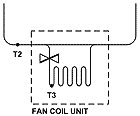What are the installation instructions for RS02 Average Room Temperature for measuring temperature in large rooms?

The following guidelines refer to Meitav-tec temperature sensor:
Maximum Length with Shield Cable - Wire length for the remote sensor can be up to 30 meters with standard thermostat cable (not less than 22AWG). If distance is longer than 30 meters, then wire must be twisted and shielded, 20-22AWG.
Cable must not pass by or be close to any High Voltage Lines or Devices.
At 200 meters, resistance may affect temperature readings by one or two degrees.

RS02 - Connection of two remote sensors (in decorative boxes) for average measuring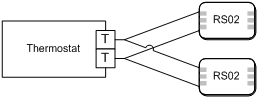Temperature ~ Resistance Characteristics

 Temp °C 7.2 10 12.8 15.6 18.3 21.1 23.9 26.7 29.4 32.2 Temp °F 45 50 55 60 65 70 75 80 85 90 Res. K 115.8 100.9 88.1 77.1 67.7 59.6 52.5 46.4 41.2 36.6

Regarding programmable thermostats, what's the difference between US and EU versions and 5-1-1 vs. 7?Programmable thermostats can be defined according to two main parameters:

1. Program Type

• USA type, day is typically divided to 2 or 4 periods, where the user programs different set points
(for Cool & Heat) , without ever turning the system off

• EU type, user can program up to 4 start times, each with a set point, and 4 stop times per day.

2. Number of Programs per week

• 5-1-1 signifies a total of 3 different programs per week; 1 for the weekdays (Monday > Friday),
1 for Saturday and 1 for Sunday

• 7-days signifies 7 programs per week; 1 for each day of the week.

RS-485 connection instructionsFollowing are main points to follow during network installation:

1. Cable Definition

One or more pairs - 120O at 1 Mhz, Made of 24# flexible twisted pairs overall foil + braid shielded and overall jacketed with a flexible PVC compound for indoor use.
Examples:
• Westernwire EG202E50 2x2x24# SFTP 120 O Digital Data Cable.
• Teldor 9392002xxx BUS-DOR RS-485/422/232 120O 2x2x24 AWG SF/UTP cable.

2. Wiring Scheme

Connect all devices by “daisy chain” topology.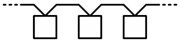3. Network Limitations

Maximum length of network should be 700 meters
Maximum units per network should be 60 units
Note: longer distance or higher capacity applicable using repeaters

4. Installation Notes

• When pulling the cable, do not use force; this may stretch the cable and distort its insulation and transmission properties.
• Do not allow the cable to kink, knot, snag, or fray when securing or rolling it out.
• Do not splice cable segments. Use continuous runs of cable from one device to another.
• Do not cinch cables ties too tightly. Do not crush cables when securing them with staples or supports. Staple by hand or use staples with depth stops.
• Maintain the color-coding of all cabling throughout your system.
• Maintain wire twisting and run the cable jacket as closely as possible to the termination point.
• Install cables and controllers to minimize the possibility of accidental contact with other, potentially hazardous and disruptive power and lighting cables.
• Do not run communication cables in the same conduit of electrical cables. If you cross an electrical cable, cross at a 90° angle.
• Do not place communication cables near other bare power cables, lightning rods, antennas, transformers, steam or hot water pipes, in any conduit, box, channel, duct or other enclosure containing power or lighting circuits of any type. Keep communication cables and controllers at least 2 meters from large inductive loads (power distribution panels, lighting ballasts, motors, etc.)

Use the attached MAC Address table to define the network addresses of the devices.

6. Termination

First and last devices in the communication line should be terminated with a 120O resistor to prevent signal reflection. When applicable, use the “End of Line” jumpers for this purpose.

7. Grounding

• Connect one end of the shield (at the end of the communication line) to a tested ground (earth). Leave the other end of shield not connected (on the air).
• Connect the shields between the devices externally (not through any terminal of the device).
• Do not connect the shields to the “ground” or “0” terminals of the devices.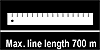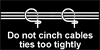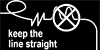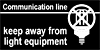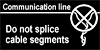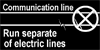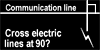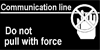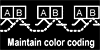8. MAC Address tableAddr. Switch position Addr. Switch position Addr. Switch position Addr. Switch position 0 NOT IN USE 64 7 128 8 192 7+8 1 1 65 1+7 129 1+8 193 1+7+8 2 2 66 2+7 130 2+8 194 2+7+8 3 1+2 67 1+2+7 131 1+2+8 195 1+2+7+8 4 3 68 3+7 132 3+8 196 3+7+8 5 1+3 69 1+3+7 133 1+3+8 197 1+3+7+8 6 2+3 70 2+3+7 134 2+3+8 198 2+3+7+8 7 1+2+3 71 1+2+3+7 135 1+2+3+8 199 1+2+3+7+8 8 4 72 4+7 136 4+8 200 4+7+8 9 1+4 73 1+4+7 137 1+4+8 201 1+4+7+8 10 2+4 74 2+4+7 138 2+4+8 202 2+4+7+8 11 1+2+4 75 1+2+4+7 139 1+2+4+8 203 1+2+4+7+8 12 3+4 76 3+4+7 140 3+4+8 204 3+4+7+8 13 1+3+4 77 1+3+4+7 141 1+3+4+8 205 1+3+4+7+8 14 2+3+4 78 2+3+4+7 142 2+3+4+8 206 2+3+4+7+8 15 1+2+3+4 79 1+2+3+4+7 143 1+2+3+4+8 207 1+2+3+4+7+8 16 5 80 5+7 144 5+8 208 5+7+8 17 1+5 81 1+5+7 145 1+5+8 209 1+5+7+8 18 2+5 82 2+5+7 146 2+5+8 210 2+5+7+8 19 1+2+5 83 1+2+5+7 147 1+2+5+8 211 1+2+5+7+8 20 3+5 84 3+5+7 148 3+5+8 212 3+5+7+8 21 1+3+5 85 1+3+5+7 149 1+3+5+8 213 1+3+5+7+8 22 2+3+5 86 2+3+5+7 150 2+3+5+8 214 2+3+5+7+8 23 1+2+3+5 87 1+2+3+5+7 151 1+2+3+5+8 215 1+2+3+5+7+8 24 4+5 88 4+5+7 152 4+5+8 216 4+5+7+8 25 1+4+5 89 1+4+5+7 153 1+4+5+8 217 1+4+5+7+8 26 2+4+5 90 2+4+5+7 154 2+4+5+8 218 2+4+5+7+8 27 1+2+4+5 91 1+2+4+5+7 155 1+2+4+5+8 219 1+2+4+5+7+8 28 3+4+5 92 3+4+5+7 156 3+4+5+8 220 3+4+5+7+8 29 1+3+4+5 93 1+3+4+5+7 157 1+3+4+5+8 221 1+3+4+5+7+8 30 2+3+4+5 94 2+3+4+5+7 158 2+3+4+5+8 222 2+3+4+5+7+8 31 1+2+3+4+5 95 1+2+3+4+5+7 159 1+2+3+4+5+8 223 1+2+3+4+5+7+8 32 6 96 6+7 160 6+8 224 6+7+8 33 1+6 97 1+6+7 161 1+6+8 225 1+6+7+8 34 2+6 98 2+6+7 162 2+6+8 226 2+6+7+8 35 1+2+6 99 1+2+6+7 163 1+2+6+8 227 1+2+6+7+8 36 3+6 100 3+6+7 164 3+6+8 228 3+6+7+8 37 1+3+6 101 1+3+6+7 165 1+3+6+8 229 1+3+6+7+8 38 2+3+6 102 2+3+6+7 166 2+3+6+8 230 2+3+6+7+8 39 1+2+3+6 103 1+2+3+6+7 167 1+2+3+6+8 231 1+2+3+6+7+8 40 4+6 104 4+6+7 168 4+6+8 232 4+6+7+8 41 1+4+6 105 1+4+6+7 169 1+4+6+8 233 1+4+6+7+8 42 2+4+6 106 2+4+6+7 170 2+4+6+8 234 2+4+6+7+8 43 1+2+4+6 107 1+2+4+6+7 171 1+2+4+6+8 235 1+2+4+6+7+8 44 3+4+6 108 3+4+6+7 172 3+4+6+8 236 3+4+6+7+8 45 1+3+4+6 109 1+3+4+6+7 173 1+3+4+6+8 237 1+3+4+6+7+8 46 2+3+4+6 110 2+3+4+6+7 174 2+3+4+6+8 238 2+3+4+6+7+8 47 1+2+3+4+6 111 1+2+3+4+6+7 175 1+2+3+4+6+8 239 1+2+3+4+6+7+8 48 5+6 112 5+6+7 176 5+6+8 240 5+6+7+8 49 1+5+6 113 1+5+6+7 177 1+5+6+8 241 1+5+6+7+8 50 2+5+6 114 2+5+6+7 178 2+5+6+8 242 2+5+6+7+8 51 1+2+5+6 115 1+2+5+6+7 179 1+2+5+6+8 243 1+2+5+6+7+8 52 3+5+6 116 3+5+6+7 180 3+5+6+8 244 3+5+6+7+8 53 1+3+5+6 117 1+3+5+6+7 181 1+3+5+6+8 245 1+3+5+6+7+8 54 2+3+5+6 118 2+3+5+6+7 182 2+3+5+6+8 246 2+3+5+6+7+8 55 1+2+3+5+6 119 1+2+3+5+6+7 183 1+2+3+5+6+8 247 1+2+3+5+6+7+8 56 4+5+6 120 4+5+6+7 184 4+5+6+8 248 4+5+6+7+8 57 1+4+5+6 121 1+4+5+6+7 185 1+4+5+6+8 249 1+4+5+6+7+8 58 2+4+5+6 122 2+4+5+6+7 186 2+4+5+6+8 250 2+4+5+6+7+8 59 1+2+4+5+6 123 1+2+4+5+6+7 187 1+2+4+5+6+8 251 1+2+4+5+6+7+8 60 3+4+5+6 124 3+4+5+6+7 188 3+4+5+6+8 252 3+4+5+6+7+8 61 1+3+4+5+6 125 1+3+4+5+6+7 189 1+3+4+5+6+8 253 1+3+4+5+6+7+8 62 2+3+4+5+6 126 2+3+4+5+6+7 190 2+3+4+5+6+8 254 2+3+4+5+6+7+8 63 1+2+3+4+5+6 127 1+2+3+4+5+6+7 191 1+2+3+4+5+6+8 255 1+2+3+4+5+6+7+8

Explanation of BACnet Device Instance Number

BACnet thermostats object list contains the Analog Value "BacnetDeviceInstanceNumber"
"Read/Write"; range 1...4194303; default value 315000 + MAC.
This Analog Value is the representation of BACnet Device object property “Object identifier”.
It may be changed via BACnet network, and BACnet router must apply it.
After updating the Device Instance Numer, the thermostat may be lost by router,
and it is necessary to rescan network to pick up the thermostat again.

Important notes:

• Thermostats in the same loop shall have different Device Instance Numbers.
• Two thermostats in different loops may have the same Device Instance Number.
• Thermostats in the same loop shall have different MAC addresses!
• The default value of the Device Instance Number can be recovered by "Restore default" operation.

•